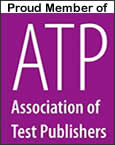UPDATED 2021

# Geometry Test Guide

Geometry, generally, is the study of size, shape and spacial relationships of lines and objects in two and three dimensions. The earliest evidence of the use of geometry is about 3000 BC in the eastern Mediterranean. Geometry was almost certainly first developed out of man’s activities in surveying, construction and early astronomy.

Today, students most often encounter geometry for the first time in 9th or 10th grade. Usually, a high school curriculum will have Algebra I given as a prerequisite to Geometry, although it is not absolutely necessary to take Algebra I first. Some curriculums will have Geometry prior to Algebra I.

Geometry curriculums obviously will vary from school district to school district and from college to college. A curriculum will proceed from introductory concepts, such as line and angles, thru intermediate concepts such as the Pythagorean Theorem, to constructing figures and determining special relationships using all principles previously learned.

The following list of activities come from a sample geometry curriculum and indicates what a student can expect to be tested on in a geometry class over the course of a year:

1. Find angle relationships, identify relationships between and among points, lines, and planes, connect geometric diagrams with algebraic representations, integrate constructions and describe, draw, and construct two-dimensional and three-dimensional figures.

2. Use angle and side relationships, use Pythagorean Theorem and its converse, use right triangle relationships such as trigonometric ratios, use properties of quadrilaterals such as classification and of other polygons.

3. Prove triangles and other polygons congruent and similar, use proportional reasoning to solve real-world problems, do indirect measurements and make scale drawings and use perimeter, circumference and area of planar regions to determine volume and surface area of solids.

4. Represent geometric figures and properties using coordinates and connect the concepts of slope, distance, and midpoint to coordinate geometry.

5. Integrate constructions, describe, draw, and construct two-dimensional and three-dimensional figures, use angle and side relationships such as triangle sum theorem, triangle inequalities, isosceles and equilateral triangle properties, altitude, and median.

6. Use right triangle relationships such as trigonometric ratios and properties of quadrilaterals such as classification; and

7. Use properties of circles, arcs, chords, central angles, inscribed angles, and concentric angles, use perimeter, circumference, and area of planar regions to determine volume and surface area of solids.